# Emitter circuit / Problem calculating peak ac amplitude

#### Tobias Hildebrandt

Joined Jan 1, 2016
44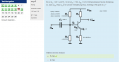I answered pretty much all questions so far, but I have no idea how to solve the next question.

Q15:
U(in): sine shaped signal, 100 Hz, amplitude = 1V. How tall is amplitude of the AC part at U(out)?
A) 4.4V B) 3.5V C) 2.3V D) 5V

Q6:
What is U(max) at U(out) if AC signal is used in the circuit.
A) 20V B) 7.5V C) 10V

Q7:
What is U(min) at U(out) if AC signal is used in circuit. (U(saturation) = 0.2V)
A) 3.5V B) 5V C) 0.2V D) 0.6V E) 7.5V

Can someone please give me a hint how to solve such a problem? Does anyone know of some good and more importantly easy to understand articles that explain that kind of problems. Finally I want to apologize for my bad English, if there something you dont understand in my questions, please feel free to ask me.

Tobias

#### Jony130

Joined Feb 17, 2009
5,457
As for Q15. Do you know anything about small-signal model? But we also can find Vout (first approximation) without small-signal analysis.
If Vin = 1V this means that the voltage at emitter will swing from 2V+1V = 3V to 2V - 1V = 1V. This well change the emitter current by
ΔIe = (2V - 1V)/400Ω = 2.5mA and since Ie≈Ic the collector current will also change by 2.5mA. So ΔVout = ΔIc * Rc = 2.5mA * 2kΩ = 5V (Voltage at collector will drop from 10V to 5V for positive half cycle)
But for this circuit we cannot do this approximation because Xc for 100Hz is 3.6kΩ ≈ Zin.
And this means that ΔVout = 5V*0.707 = 3.5V

Q6- Vmax is reach when BJT is cut-off
Q7 - Vmin is reach when transistor enters saturation region

http://users.ece.gatech.edu/mleach/ece3050/notes/bjt/bjtclipsu08.pdf
http://eelinux.ee.usm.maine.edu/courses/ele343/343 Class Notes-Handouts/Lecture Notes/2. BJT Amps for Undistorted Swing/7.BJT Amps. for Undistorted VoltageSwing-X.pdf
Also you cannot buy 400Ω; 4.6kΩ and 30.8kΩ resistors, also it is impassible to buy a 442nF capacitor. So, why you do not use a standard resistor values for example from a 5% tolerance resistor E24 sequence, and E6 for capacitors ?

Last edited:
•anhnha and Tobias Hildebrandt

#### MrAl

Joined Jun 17, 2014
10,587
Hi,

But isnt this just a homework problem, where they use all sorts of unrealistic values for example problems?

Also, a quick estimate of the transistor gain is Rc/Re, which in this case is R3/R4 which is 2000/400, which comes out to a gain of 5.
Now if the base voltage changed by 1v the output would change by 1*5=5v that's true. But the input voltage to the circuit is 1v, not the base voltage, and there are components between the input and the base. This means we probably have to figure in the effect of those components as well.

Calculating the input to base attenuation due to the input cap and input impedance, we get an attenuation factor of about 0.707 and when multiplied by 1 we get of course 0.707 again. Multiplying this by the gain of the transistor of 5, we get something fairly close to 3.5v. If the input was in peak volts (1v peak) then the output in peak volts would be 3.5v peak.

I am not sure if this helps or not, but here is a link i found that is right here on this forum:

Last edited:
•anhnha and Tobias Hildebrandt

#### Tobias Hildebrandt

Joined Jan 1, 2016
44
Also you cannot buy 400Ω; 4.6kΩ and 30.8kΩ resistors, also it is impassible to buy a 442nF capacitor. So, why you do not use a standard resistor values for example from a 5% tolerance resistor E24 sequence, and E6 for capacitors ?
I am pretty sure Prof. Dr. Best wanted to provide us with the "correct" result from the equations. In one of the exam questions, he actually makes you pick the fitting transistors that match your design. This Moodle test is just there to familiarize yourself with the concepts of designing a circuit.

#### Jony130

Joined Feb 17, 2009
5,457
OK I see. Is this is some kind of a online test ?? And have you already find the answer for Q6/Q7 ?

#### Tobias Hildebrandt

Joined Jan 1, 2016
44
OK I see. Is this is some kind of a online test ?? And have you already find the answer for Q6/Q7 ?
The online learning platform is called Moodle, it gives Professors the possibility to be more interactive with the students. Professors can make you take tests or you can drop of your homework through Moodle. In theory it is a good idea, because it can provide feedback to you and the Profs, but it really depends on how much time the Profs want to invest in it. This Prof. actually uses it rather extensively, he puts all of his lecture nodes and old exams online through this platform.

Anyway, right now I am working on Q13. I calculated for Xc the same value as you (1/(2*Pi*100*442*10^(-9)=3.6kOhm), but I am not sure how to calculate the "attenuation factor"? Tried to find some answers in the forum, but without success.

The other two questions I will work on when I am done with Q13.

Tobias

#### Jony130

Joined Feb 17, 2009
5,457
I calculated for Xc the same value as you (1/(2*Pi*100*442*10^(-9)=3.6kOhm), but I am not sure how to calculate the "attenuation factor"? Tried to find some answers in the forum, but without success.
Have you study RC circuit in frequency domain ? For example a high pass filter ?
And notice that Xc = Zin and every old hand in electronics knows that for this case, the attenuation factor is equal to Zin/(Xc + Zin) = 1/√2 ≈ 0.707

#### Tobias Hildebrandt

Joined Jan 1, 2016
44
Q13. Is the Root mean squared amplitude = attenuation factor?

So, the only thing I have to do is to 1/sqrt(2) * signal peak amplitude * amplification(is this the right word? ) ?

In one of your links the postive peak output voltage is given by I(c)*R(c)//R(l).

Our circuit does not have a load, and multiplying I(c)*R(c)=10V is not getting me anywhere. Hmm, bugger. Any ideas?

#### Jony130

Joined Feb 17, 2009
5,457
Q13. Is the Root mean squared amplitude = attenuation factor?

So, the only thing I have to do is to 1/sqrt(2) * signal peak amplitude * amplification(is this the right word? ) ?
Yes , the attenuation factor for the case where Xc = Zin is equal to $$\frac{1}{\sqrt{2}}=0.707$$
In general case Xc together with Zin form a frequency dependent voltage divider.

$$\frac{Vout}{Vin} = \frac{Zin}{\sqrt{Zin^2 + Xc^2}}$$

In one of your links the postive peak output voltage is given by I(c)*R(c)//R(l).

Our circuit does not have a load, and multiplying I(c)*R(c)=10V is not getting me anywhere. Hmm, bugger. Any ideas?
Try to think logically. Vout_max occurs when BJT is cut-off, this means Ic = 0A.
So, the voltage at Vout is ??

•Tobias Hildebrandt

#### MrAl

Joined Jun 17, 2014
10,587
Hello again,

In many of these circuits they use some kind of approximation somewhere. For example in this circuit we see that they approximate the output as 1/2 of the supply voltage Ucc, and that sets the current in R3, and that sets the current in R4 with the approximation IC=IE. That's where the 2v emitter voltage comes from, and they seem to ignore re which is small anyway relative to external R4.
This leads to 2.6v at the base, Ubb.

Without that approximation however, the output bias point comes out to:
Uout=(Beta*Ucc*R2*R4+Ucc*R2*R4+Beta*Ucc*R1*R4+Ucc*R1*R4
-Beta*Ucc*R2*R3+Beta*Ube*R2*R3+Beta*Ube*R1*R3+Ucc*R1*R2)/(Beta*
R2*R4+R2*R4+Beta*R1*R4+R1*R4+R1*R2)

where R4 above actually equals R4+re but you could probably ignore re.
This works out to slightly above 11 volts DC, with no input signal.
That means the most positive excursion is 11+3.5 and the most negative is 11-3.5, but since they used the approximation Ic=Ie that means they probably want you to assume that the output is biased at 10v, so this would be 10+3.5 and 10-3.5 for the two excursions.

The transfer function for input voltage to output collector current is:
Ic/Uin=
(Beta*(s*C1*E1*R1*R2-s*Ube*C1*R1*R2+Ucc*R2-Ube*R2-Ube*R1))/
(Beta*s*C1*R1*R2*R4+s*C1*R1*R2*R4+Beta*R2*R4+R2*R4+Beta*R1*
R4+R1*R4+R1*R2)

Once you have the transfer function you can calculate just about anything. To calculate the AC output voltage you can multiply that by R3.

We could also transform this into the time domain and plot the output voltage vs time.

If you dont know how to find a transfer function maybe you could look into this or ask your instructor for help. The reason i say this is because you can calculate a lot about any circuit once you have that kind of equation, and it's usually just a little more work.
The transfer function can be calculated using an analysis method such as Nodal Analysis.

Last edited:
•Tobias Hildebrandt

#### Tobias Hildebrandt

Joined Jan 1, 2016
44
I bought a new book about transistors, but I still can't find an answer to Q7.

U(CEsat) = 0.2 What is the smallest possible value at U(out) if the circuit receives an AC signal?

a) 3.5V b) 5V c) 0.2V d) 0.6V e) 7.5V

Did I even understand the question correctly? There is one smallest U(out) regardless of U(in) AC signal. In other words, it does not matter if I have 5V or 50V input signal it will always lead to one value?

I appreciate all the comments! Thanks again.

Is there a fairly simple way to calculate it?

#### Jony130

Joined Feb 17, 2009
5,457
In Q6 and Q7 they ask you about the maximum voltage swing at the output node.
For example, without RE resistor the voltage at the output can swing from 10V to 20V for positive half cycle (transistor cut-off) and for the negative half cycle the voltage at the output can swing from 10V to Vce(sat) = 0.2V (transistor enter saturation region).
Therefor V(max) = 20V and V(min) = 0.2VAnd this is the maximum output voltage swing which occurs when Vin ≥ Vmax_swing/Voltage_gain = 10V/5 = 2V, So any input voltage larger then 2Vpeak will cause "clipping" at the output.

But in your amplifier you have a RE resistor and DC voltage at this resistor is 2V. Also at the same time the voltage at the emitter will also change in the "rhythm" of a input signal. And this drop across Re resistor increases the saturation voltage and in the same time increases V(min).
Try once again analysis this circuitThe voltage gain is Rc/Re = 3V/V and Vcc = 12V; Vc = 6V (red plot ); Ve = 2V (green plot) ; Vinput = 1V (blue plot).
Also notice that for Vin = 1V the voltage at emitter also swing by 1V(from 2V to 3V and from 2V down to 1V).
And I hope that you can see that Vout_max = 12V but Vout_(min) = 3V. Because any input voltage larger than 1V will cause BJT to saturate and clipping (Ve - want to be larger than 3V, but at the same time Vc want to be smaller than 3V).
So for this circuit V(max) = 12V and V(min) = 3V = 12V*Re/(Re+Rc) for Vce(sat) = 0V.

Back to your amplifier and Q7.
You can find V(min) if you solve for Vout for this equivalent circuit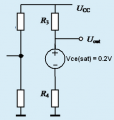#### Tobias Hildebrandt

Joined Jan 1, 2016
44
I am thankful for your hard work and very frustrated about my own limitations to understand circuits!

Anyway, let me try a more structured approach and ask my questions one by one.

Using your formula, I came up with the 3.5 V result, which appeared to be correct.

V(min) = 20V * 400Ohm/(2000Ohm+400Ohm) roughly 3.3V.

Here my questions for Q7:
- Is this formula correct and can I use it for all emitter circuits?
- Do I just add the voltage drop to my result?
- Your equivilant circuit does confuse me. I am puzzled by all the ins and outs. So I am not really sure how to calculate it. R3 and R4 appear to be parallel to each other, but what happens with Vce(sat)?

Q13.
I looked over your answers for Q13 again and the formula you provided is very straightforward. I am still not 100% sure, when I can use the "attenuation factor" and when I can not use it.

Questions Q13
-Can you please shine some light on it, when the attenuation factor can be used.
-When I calculated the capacitive reactance (Xc), using the 100Hz frequency, than it gave me the exact result. Is it enough, just to calculate Xc or is this coincidence? (Problem if frequency is not given, I can't calculate Xc. Do you know what I mean?)
-V(out)/V(in) = Z(in) /sqrt(Z(in)^2+X(c)^2) . This formula provides me with the same problem, I need to make assumptions about frequency.
In other words when can I use 1/sqrt(2) and what to do if frequency is not given in the question?

Q16 is a NEW question.
What phase shift does the output signal have compared to the input signal at a frequency of 100Hz? (Comment: This is where I found the 100Hz; because this information was provided after Q13 I am pretty sure, Q13 can be solved without using this information)

Prof. Dr. Best provided the following possible answers:
a) inverted (180 degree difference) b) Phase is lagging by 45 degrees c) Phase is lagging by 135 d) Phase is "leading" by 45 degrees e) no phase shift

My solution was wrong. This is what I did. I assumed that I can use the formulas for a high pass filter (f(c)= 1/(2*pi*R*C)). This assumption proved to be wrong. The result of 78.28 degrees was not an option. According to Moodle the correct answer is phase lagging by 135 degrees.

Question.
If it is not a highpass filter what formulas do I use to calculate the phase shift?

Thanks again for all your help!!!
Tobias

#### Tobias Hildebrandt

Joined Jan 1, 2016
44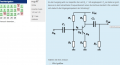I hope you see, I dont bother you for all the questions! I am trying hard to understand this, but I am struggling here and there.

New Question:
Q20
What is the lowest possible signal at U(out), if you put an AC signal at U(in) (While having an unchanged voltage across C2? (Comment: the voltage was calculated in Q18 and I got the right answer. The answer was 10V).
Not even a vague idea where to start!

Q21
U(in) sign shaped signal.Amplitude 1V. What amplitude has the AC at U(out)?

Why?

#### Tobias Hildebrandt

Joined Jan 1, 2016
44
Anyway, I just wanted to add this for your information. We have exams in three weeks and I do this Moodle test to prepare for the exam. So I am not so much interested in getting the right answer, but much more interested why the circuit behaves in a certain way. Other Professors at our university recycle old exam questions, but not Prof. Dr. Best. He writes every semester a totally new exam, so understanding the circuits is of utmost importance.

#### Jony130

Joined Feb 17, 2009
5,457
- Is this formula correct and can I use it for all emitter circuits?
No you can't

- Do I just add the voltage drop to my result?
What ?

- Your equivalent circuit does confuse me. I am puzzled by all the ins and outs. So I am not really sure how to calculate it. R3 and R4 appear to be parallel to each other, but what happens with Vce(sat)?
Try again with this equivalent circuit circuit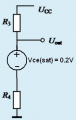I looked over your answers for Q13 again and the formula you provided is very straightforward. I am still not 100% sure, when I can use the "attenuation factor" and when I can not use it.
Also, if you do not have any information about the signal frequency and capacitor value, it is impossible to solve for "attenuation factor".
But "attenuation factor" is equal 0.707 only at frequency when Xc = R (F = 1/(2*pi*R*C))
And without this type of a information we usually assume that Xc = 0Ω.
If it is not a highpass filter what formulas do I use to calculate the phase shift?
The input capacitor together with Zin form a high pass filter. Also, can you tell me how did you get this result "78.28 degrees"? Haven't you study a high pass filter before?
At frequency when Xc=R the phase shift is +45° but amplifier (alone) invert the phase by 180 and this is why the answer is -135°.

Q20
What is the lowest possible signal at U(out), if you put an AC signal at U(in) (While having an unchanged voltage across C2? (Comment: the voltage was calculated in Q18 and I got the right answer. The answer was 10V).
Not even a vague idea where to start!
Try this circuit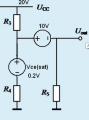U(in) sign shaped signal.Amplitude 1V. What amplitude has the AC at U(out)?

Can you solve for the voltage gain ??

#### Tobias Hildebrandt

Joined Jan 1, 2016
44
1) Quote:
"- Do I just add the voltage drop to my result?
What ?"

My answer: V(min) = 20V * 400Ω/(2000Ω+400Ω) ≈ 3.3V + 0.2V = 3.5V (Comment: Voltage drop. This is what I meant when I asked if I just add the Voltage drop my result).

2) Quote:
Try again with this equivalent circuit

I see. So it is just a good old fashioned voltage divider. It gets me exactly the same equation as above. Good. (When I played around with the formula, I wanted to add 0.2 to U(2). I am still not 100% sure why I have to subtract it and not add it)

U(2) - 0.2V = 20V * 400Ω/(2000Ω+400Ω)

3) Quote:
The input capacitor together with Zin form a high pass filter. Also, can you tell me how did you get this result "78.28 degrees"? Haven't you study a high pass filter before?
At frequency when Xc=R the phase shift is +45° but amplifier (alone) invert the phase by 180 and this is why the answer is -135°.

Yes, we studied high pass filters before. I got the 78.28 degrees by using the value of R2, that was my mistake. When I used Z(in) I got 45 degrees as well. I have to invert the phase because it is an emitter circuit, if it would be a emitter-follower circuit I could just use the 45 degrees and get the correct answer, is this assessment correct?

Quote: Can you solve for the voltage gain ??

This is my attempt it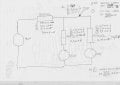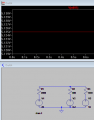Well, my calculations gave me 4.88V as you can see above. How can I tell that it is -4.88V? Does that have sth. to do with Kirchhoff?

When I used LTSpice to verify my result, I got 5.25V, but this puzzles me. Doesnt the voltage drop across a resistor? So if you have on the one side of the transistor 10V and on the other 5.25V, WELL, how does the 4.88V fit into this scenario???

#### Tobias Hildebrandt

Joined Jan 1, 2016
44
Wonderful. I think I solved this question:

U(in) sin shaped signal.Amplitude 1V. What amplitude has the AC at U(out)?

Can you solve for the voltage gain ??

Found this in my book: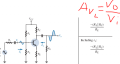V(i) = 0.707V
1kΩ parallel 2kΩ / 400Ω = 1.666.
If I multiply the numbers with each other I should get V(o). Using this method I got the 'correct' value of 1.17V, BUT what happens to the minus sign infront of the equation? It clearly says -(R(l) parallel R(c))/R(e).
Tobias

#### Jony130

Joined Feb 17, 2009
5,457
My answer: V(min) = 20V * 400Ω/(2000Ω+400Ω) ≈ 3.3V + 0.2V = 3.5V (Comment: Voltage drop. This is what I meant when I asked if I just add the Voltage drop my result).
From KVL (II Kirchhoff's law) we have:
Vcc = Ic*R3 + Vce(sat)*Ic*R4

And if we solve for Ic we have

Ic = (Vcc - Vce)/(R3 + R4)

But at the same time Vout = Ic*R4+Vce(sat) = (Vcc - Vce(sat))*R4/(R3+R4) + Vce(sat) = 3.5V

, if it would be a emitter-follower circuit I could just use the 45 degrees and get the correct answer, is this assessment correct?
Yes, you are right.
Well, my calculations gave me 4.88V as you can see above. How can I tell that it is -4.88V? Does that have sth. to do with Kirchhoff?
When I used LTSpice to verify my result, I got 5.25V, but this puzzles me. Doesnt the voltage drop across a resistor? So if you have on the one side of the transistor 10V and on the other 5.25V, WELL, how does the 4.88V fit into this scenario???
Vc = 2.5V + 0.125V + 2.5V = 5.125V

The original circuit looks like this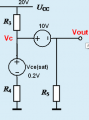For this circuit it is clear that Vout = Vc - 10V and
Vc = Vcc * (R4||R5)/(R3 + R4||R5) + Vce * (R3||R5)/(R4 + R3||R5) + Vcap* (R3||R4)/(R5 + R3||R4) = 5.125V

therefore

Vout =
5.125V - 10V = -4.875V
Using this method I got the 'correct' value of 1.17V, BUT what happens to the minus sign infront of the equation? It clearly says -(R(l) parallel R(c))/R(e).
Yes, the voltage gain for CE amplifier is negative. But we usually forget about this "minus" sign. Because this "minus" only inform us that our amplifier output is 180° out of phase with its input nothing more.
And voltage gain is equal to Av = - (Rc||RL)/(Re + r'e)

Last edited: